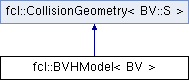FCL  0.6.0 Flexible Collision Library
fcl::BVHModel< BV > Class Template Reference

A class describing the bounding hierarchy of a mesh model or a point cloud model (which is viewed as a degraded version of mesh) More...

`#include <BVH_model.h>`

Inheritance diagram for fcl::BVHModel< BV >:## Public Types

using S = typename BV::S

## Public Member Functions

BVHModelType getModelType () const
Model type described by the instance.

BVHModel ()
Constructing an empty BVH.

BVHModel (const BVHModel &other)
copy from another BVH

~BVHModel ()
deconstruction, delete mesh data related.

const BVNode< BV > & getBV (int id) const
We provide getBV() and getNumBVs() because BVH may be compressed (in future), so we must provide some flexibility here. More...

BVNode< BV > & getBV (int id)
Access the bv giving the its index.

int getNumBVs () const
Get the number of bv in the BVH.

OBJECT_TYPE getObjectType () const override
Get the object type: it is a BVH.

NODE_TYPE getNodeType () const override
Get the BV type: default is unknown.

void computeLocalAABB () override
Compute the AABB for the BVH, used for broad-phase collision.

int beginModel (int num_tris=0, int num_vertices=0)
Begin a new BVH model.

int addVertex (const Vector3< S > &p)
Add one point in the new BVH model.

int addTriangle (const Vector3< S > &p1, const Vector3< S > &p2, const Vector3< S > &p3)
Add one triangle in the new BVH model.

int addSubModel (const std::vector< Vector3< S >> &ps, const std::vector< Triangle > &ts)
Add a set of triangles in the new BVH model.

int addSubModel (const std::vector< Vector3< S >> &ps)
Add a set of points in the new BVH model.

int endModel ()
End BVH model construction, will build the bounding volume hierarchy.

int beginReplaceModel ()
Replace the geometry information of current frame (i.e. should have the same mesh topology with the previous frame)

int replaceVertex (const Vector3< S > &p)
Replace one point in the old BVH model.

int replaceTriangle (const Vector3< S > &p1, const Vector3< S > &p2, const Vector3< S > &p3)
Replace one triangle in the old BVH model.

int replaceSubModel (const std::vector< Vector3< S >> &ps)
Replace a set of points in the old BVH model.

int endReplaceModel (bool refit=true, bool bottomup=true)
End BVH model replacement, will also refit or rebuild the bounding volume hierarchy.

int beginUpdateModel ()
Replace the geometry information of current frame (i.e. should have the same mesh topology with the previous frame). The current frame will be saved as the previous frame in prev_vertices.

int updateVertex (const Vector3< S > &p)
Update one point in the old BVH model.

int updateTriangle (const Vector3< S > &p1, const Vector3< S > &p2, const Vector3< S > &p3)
Update one triangle in the old BVH model.

int updateSubModel (const std::vector< Vector3< S >> &ps)
Update a set of points in the old BVH model.

int endUpdateModel (bool refit=true, bool bottomup=true)
End BVH model update, will also refit or rebuild the bounding volume hierarchy.

int memUsage (int msg) const
Check the number of memory used.

void makeParentRelative ()
This is a special acceleration: BVH_model default stores the BV's transform in world coordinate. However, we can also store each BV's transform related to its parent BV node. When traversing the BVH, this can save one matrix transformation.

Vector3< S > computeCOM () const override
compute center of mass

computeVolume () const override
compute the volume

Matrix3< S > computeMomentofInertia () const override
compute the inertia matrix, related to the originPublic Member Functions inherited from fcl::CollisionGeometry< BV::S >
void * getUserData () const
get user data in geometry

void setUserData (void *data)
set user data in geometry

bool isOccupied () const
whether the object is completely occupied

bool isFree () const
whether the object is completely free

bool isUncertain () const
whether the object has some uncertainty

virtual Matrix3< BV::S > computeMomentofInertiaRelatedToCOM () const
compute the inertia matrix, related to the com

## Public Attributes

Vector3< S > * vertices
Geometry point data.

Triangletri_indices
Geometry triangle index data, will be nullptr for point clouds.

Vector3< S > * prev_vertices
Geometry point data in previous frame.

int num_tris
Number of triangles.

int num_vertices
Number of points.

BVHBuildState build_state
The state of BVH building process.

std::shared_ptr< detail::BVSplitterBase< BV > > bv_splitter
Split rule to split one BV node into two children.

std::shared_ptr< detail::BVFitterBase< BV > > bv_fitter
Fitting rule to fit a BV node to a set of geometry primitives.Public Attributes inherited from fcl::CollisionGeometry< BV::S >
Vector3< BV::S > aabb_center
AABB center in local coordinate.

AABB< BV::S > aabb_local
AABB in local coordinate, used for tight AABB when only translation transform.

void * user_data
pointer to user defined data specific to this object

BV::S cost_density
collision cost for unit volume

BV::S threshold_occupied
threshold for occupied ( >= is occupied)

BV::S threshold_free

## Friends

template<typename , typename >
struct MakeParentRelativeRecurseImpl

## Detailed Description

### template<typename BV> class fcl::BVHModel< BV >

A class describing the bounding hierarchy of a mesh model or a point cloud model (which is viewed as a degraded version of mesh)

## Member Function Documentation

template<typename BV >
 const BVNode< BV > & fcl::BVHModel< BV >::getBV ( int id ) const

We provide getBV() and getNumBVs() because BVH may be compressed (in future), so we must provide some flexibility here.

Access the bv giving the its index

The documentation for this class was generated from the following files: